NCERT Solutions for Class 7 Maths Chapter 6 The Triangle and its Properties Ex 6.5 are part of NCERT Solutions for Class 7 Maths. Here we have given NCERT Solutions for Class 7 Maths Chapter 6 The Triangle and its Properties Ex 6.5.

 Board CBSE Textbook NCERT Class Class 7 Subject Maths Chapter Chapter 6 Chapter Name The Triangle and its Properties Exercise Ex 6.5 Number of Questions Solved 8 Category NCERT Solutions

## NCERT Solutions for Class 7 Maths Chapter 6 The Triangle and its Properties Ex 6.5

Question 1.
PQR is a triangle right-angled at P. If PQ = 10 cm and PR = 24 cm, find QR.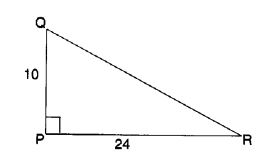Solution:
QR2 = 102 + 242 By Pythagoras Property
⇒ = 100 + 576 = 676
⇒ QR = 26 cm.

Question 2.
ABC is a triangle right-angled at C. If AB – 25 cm and AC = 7 cm, find BC.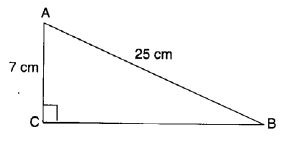Solution:
AC2 + BC2 = AB2 By Pythagoras Property
⇒ 72 + BC2 = 252
⇒ 49 + BC2 = 625
⇒ BC2 = 625 – 49
⇒ BC2 = 576
⇒ BC = 24 cm.

Question 3.
A 15 m long ladder reached a window 12 m high from the ground on placing it against a wall at a distance a. Find the distance of the foot of the ladder from the wall.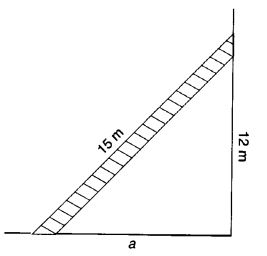Solution:
Let the distance of the foot of the ladder from the wall be a m. Then,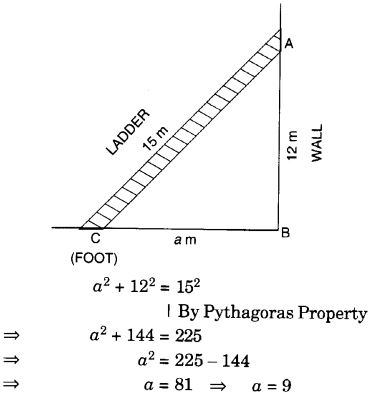Hence, the distance of the foot of the ladder from the wall is 9 m.

Question 4.
Which of the following can be the sides of a right triangle ?

1. 2.5 cm, 6.5 cm, 6 cm.
2. 2 cm, 2 cm, 5 cm.
3. 1.5 cm, 2 cm, 2.5 cm.

In the case of right-angled triangles, identify the right angles.
Solution:
1. 2.5 cm, 6.5 cm, 6 cm We see that
(2.5)2 + 62 = 6.25 + 36 = 42.25 = (6.5)2
Therefore, the given lengths can be the sides of a right triangle. Also, the angle between the lengths, 2.5 cm and 6 cm is a right angle.

2. 2 cm, 2 cm, 5 cm
∵ 2 + 2 = 4 $$\ngtr$$ 5
∴ The given lengths cannot be the sides of a triangle
The sum of the lengths of any two sides of a triangle is greater than the third side

3. 1.5 cm, 2 cm, 2.5 cm We find that
1.52 + 22 = 2.25 + 4 = 6.25 = 2.52
Therefore, the given lengths can be the sides of a right triangle.
Also, the angle between the lengths 1.5 cm and 2 cm is a right angle.

Question 5.
A tree is broken at a height of 5 m from the ground and its top touches the ground at a distance of 12 m from the base of the tree. Find the original height of the tree.
Solution:
AC = CD Given
In right angled triangle DBC, DC2 = BC2 + BD2
by Pythagoras Property = 52 + 122 = 25 + 144 = 169⇒ DC = 13 ⇒ AC = 13
⇒ AB = AC + BC = 13 + 5 = 18
Therefore, the original height of the tree = 18 m.

Question 6.
Angles Q and R of a ∆ PQR are 25° and 65°. Write which of the following is true:(i) PQ2 + QR2 = RP2
(ii) PQ2 + RP2 = QR2
(iii) RP2 + QR2 = PQ2
Solution:
(ii) PQ2 + RP2 = QR2 is true.

Question 7.
Find the perimeter of the rectangle whose length is 40 cm and a diagonal is 41 cm.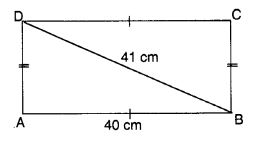Solution:
In right-angled triangle DAB, AB2 + AD2 = BD2
⇒ 402 + AD2 = 412 ⇒ AD2 = 412 – 402
⇒ AD2 = 1681 – 1600
∴ Perimeter of the rectangle = 2(AB + AD) = 2(40 + 9) = 2(49) = 98 cm
Hence, the perimeter of the rectangle is 98 cm.

Question 8.
The diagonals of a rhombus measure 16 cm and 30 cm. Find its perimeter.Solution:
Let ABCD be a rhombus whose diagonals BD and AC are of lengths 16 cm and 30 cm respectively.
Let the diagonals BD and AC intersect each other at O.
Since the diagonals of a rhombus bisect each other at right angles. Therefore
BO = OD = 8 cm,
AO = OC = 15 cm,
∠AOB = ∠BOC
= ∠COD = ∠DOA = 90°
In right-angled triangle AOB.
AB2 = OA2 + OB2
By Pythagoras Property
⇒ AB2 = 152 + 82
⇒ AB2 = 225 + 64
⇒ AB2 = 289
⇒ AB = 17cm
Therefore, perimeter of the rhombus ABCD = 4 side = 4 AB = 4 × 17 cm = 68 cm
Hence, the perimeter of the rhombus is 68 cm.

We hope the NCERT Solutions for Class 7 Maths Chapter 6 The Triangle and its Properties Ex 6.5 help you. If you have any query regarding NCERT Solutions for Class 7 Maths Chapter 6 The Triangle and its Properties Ex 6.5, drop a comment below and we will get back to you at the earliest.